# Chemistry Practice MCQs for Class 12 – Chapter 2, 10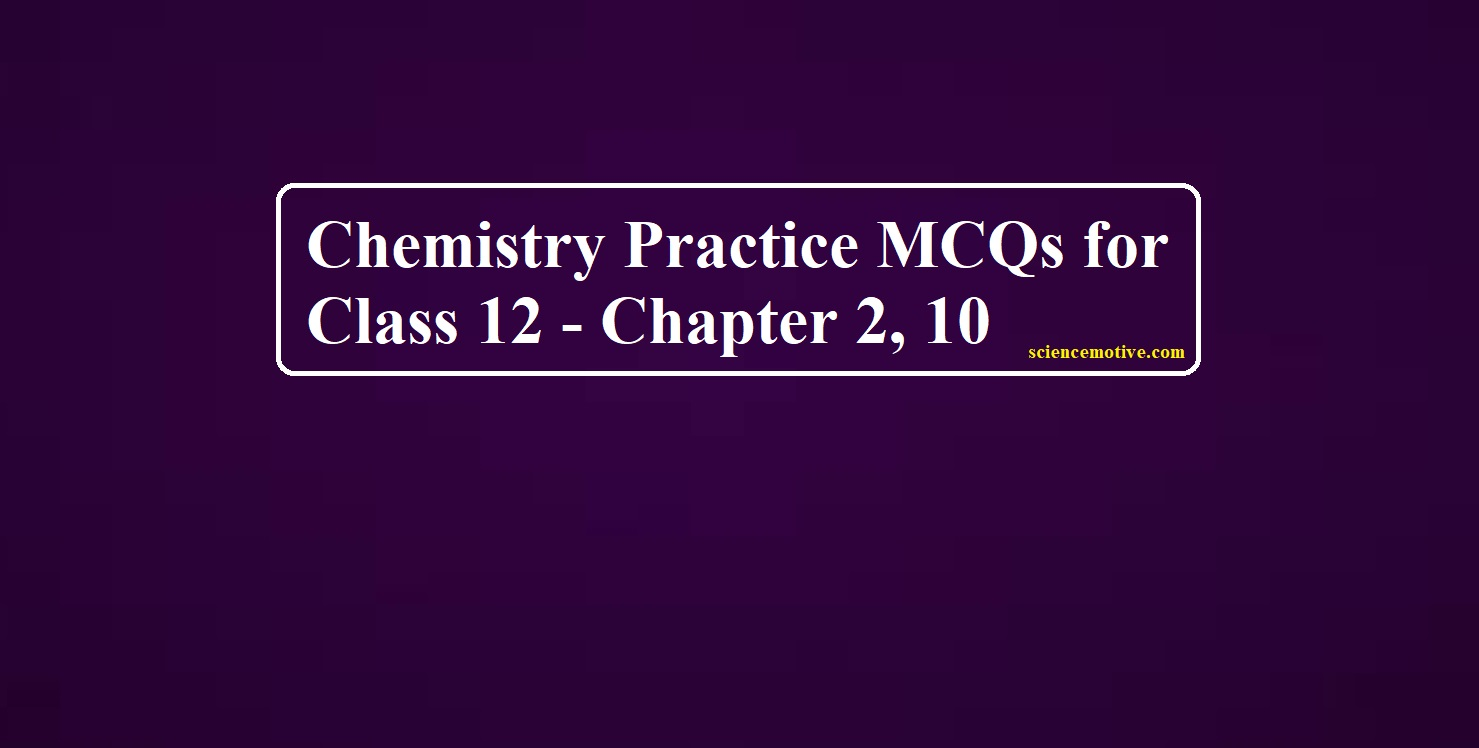# Chemistry Practice MCQs for Class 12

Chemistry Practice MCQs for Class 12

1. NaCl is 100 % ionized in a 0.01 molal aqueous solution. Hence the ratio of elevation in boiling point to molal elevation constant is:
(a) 0.01
(b) 0.015
(c) 0.0175
(d) 0.02

2. Which has the highest boiling point:
(a) 0.1 M Sodium sulphate
(b) 0.1 M glucose
(c) 0.1 M Magnesium chloride
(d) 0.1 M Aluminium nitrate

3. What is the effect of the addition of sugar on the boiling and freezing point of water
(a) Both Boiling Point and Freezing Point Increases
(b) Both Boiling Point and Freezing Point Decreases
(c) Boiling Point Increases and Freezing Point Decreases
(d) Boiling Point Decreases and Freezing Point Increases

4. Which of the following aqueous molal solution have the highest freezing point
(a) Urea
(b) Barium chloride
(c) Potassium bromide
(d) Aluminium sulphate

5. Which will show maximum depression in freezing point when concentration is 0.1 M
(a) NaCl
(b) Urea
(c) Glucose
(d) Potassium sulphate

6. Colligative properties of the solution depend on
(a) Nature of the solution
(b) Nature of the solute particles
(c) Concentration of solute particles
(d) All of these

7. Isotonic solutions must have the same
(a) Osmotic pressure
(b) Density
(c) Elevation in boiling point
(d) Depression in freezing point

8. Osmosis is
(a) Flow of solute particles, from solution to solvent through SPM
(b) Flow of solvent particles, from solvent to solution through SPM
(c) Flow of solvent particles, from solution to solvent through SPM
(d) Flow of solvent particles, from high concentrated solution to low concentrated solution through SPM

9. The compound whose 0.1 M solution has maximum osmotic pressure at the given temperature will be
(a) Calcium chloride
(b) KCl
(c) Glucose
(d) Urea

10. Observe the following figure and state which is a correct statement.

(a) Water flows from freshwater to salt waterside
(b) Water flows from saltwater to fresh waterside
(c) There is no flow of water
(d) None of the above

Chemistry Practice MCQs for Class 12

11. The osmotic pressure of the solution can be increased by
(a) Increasing temperature of the solution
(b) Decreasing temperature of the solution
(c) Increasing the volume of the solution
(d) Diluting the solution

12. If liquid A and B form ideal solutions
(a) The enthalpy of mixing is zero
(b) The entropy of mixing is zero
(c) The free energy of mixing is zero
(d) The free energy as well as the entropy of mixing are zero

Directions: In the following questions, A statement of Assertion (A) is followed by a statement of Reason (R). Mark the correct choice as.
(A) Both A and R are true and R is the correct explanation of A
(B) Both A and R are true but R is NOT the correct explanation of A
(C) A is true but R is false
(D) A is false and R is True

13. Assertion: When NaCl is added to water a depression in the freezing point is observed.
Reason: The lowering of vapour pressure of a solution causes depression in the freezing point

14. Assertion: Osmotic pressure is a colligative property.
Reason: Osmotic pressure depends on the nature of solute molecules

15. Assertion: 0.1 M solution of KCl has great osmotic pressure than 0.1 M solution of glucose at the same temperature.
Reason (R): In solution, KCl dissociates to produce more number of particles.

16. Assertion: Phosphorus chlorides (tri and penta) are preferred over thionyl chloride for the preparation of alkyl chlorides from alcohols.
Reason: Phosphorus chlorides give pure alkyl halides.

17. Assertion: The presence of a nitro group at ortho or para position increases the reactivity of haloarenes towards nucleophilic substitution.
Reason: Nitro group, being an electron-withdrawing group decreases the electron density over the benzene ring.

18. Assertion: In monohaloarenes, further electrophilic substitution occurs at ortho and para positions.
Reason (R): Halogen atom is a ring deactivator. R

19. Assertion: It is difficult to replace chlorine with – OH in chlorobenzene in comparison to that in chloroethane.
Reason: Carbon-chlorine (C—Cl) bond in chlorobenzene has a partial double bond character due to resonance.

Chemistry Practice MCQs for Class 12

The boiling point or freezing point of the liquid solution would be affected by the dissolved solids in the liquid phase. A soluble solid in solution has the effect of raising its boiling point and depressing its freezing point. The addition of non-volatile substances to a solvent decreases the vapor pressure and the added solute particles affect the formation of pure solvent crystals. According to many types of research the decrease in freezing point directly correlated to the concentration of solutes dissolved in the solvent. This phenomenon is expressed as freezing point depression and it is useful for several applications such as freeze concentration of liquid food and to find the molar mass of an unknown solute in the solution. Freeze concentration is a high-quality liquid food concentration method where water is removed by forming ice crystals. This is done by cooling the liquid food below the freezing point of the solution. The freezing point depression is referred to as a colligative property and it is proportional to the molar concentration of the solution (m), along with vapor pressure lowering, boiling point elevation, and osmotic pressure. These are physical characteristics of solutions that depend only on the identity of the solvent and the concentration of the solute. The characters are not depending on the solute’s identity.
i) When a non-volatile solid is added to pure water it will
(a) Boil above 100°C and freeze above 0°C
(b) Boil below 100°C and freeze above 0°C
(c) Boil above 100°C and freeze below 0°C
(d) Boil below 100°C and freeze below 0°C

ii) Colligative properties are
(a) Dependent only on the concentration of the solute and independent of the solvents and solute’s identity.
(b) Dependent only on the identity of the solute and the concentration of the solute and independent of the solvent’s identity.
(c) Dependent on the identity of the solvent and solute and thus on the concentration of the solute.
(d) Dependent only on the identity of the solvent and the concentration of the solute and independent of the solute’s identity.

iii) Assume three samples of juices A, B and C have glucose as the only sugar present in them. The concentration of samples A, B, and C are 0.1M, .5M, and 0.2 M respectively. The freezing point will be highest for the fruit juice
(a) A
(b) B
(c) C
(d) All have the same freezing point.

iv) Identify which of the following is a colligative property:
(a) Freezing Point
(b) Boiling Point
(c) Osmotic Pressure
(d) All of The Above

Chemistry Practice MCQs for Class 12

21. The order of reactivity of following alcohols with halogen acids is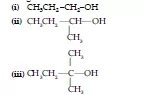(a) (i) > (ii) > (iii)
(b) (iii) > (ii) > (i)
(c) (ii) > (i) > (iii)
(d) (i) > (iii) > (ii)

22. Which of the following alcohols will yield the corresponding alkyl chloride on reaction with concentrated HCl at room temperature?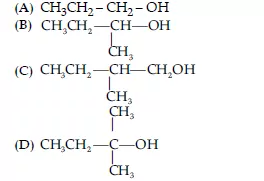23. Arrange the following compounds in increasing order of their boiling points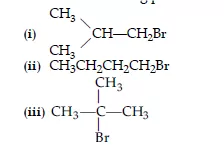(a) (ii) < (i) < (iii)
(b) (i) < (ii) < (iii)
(c) (iii) < (i) < (ii)
(d) (iii) < (ii) < (i)

24. The conversion of an alkyl halide into alcohol by aqueous NaOH is classified as
(a) A dehydrohalogenation reaction
(b) A substitution reaction
(d) A dehydration reaction

25. Which of the following alkyl halides will undergo SN1 reaction most readily?
(a) (CH3)3C—F
(b) (CH3)3C—Cl
(c) (CH3)3C—Br
(d) (CH3)3C—I

26. Which reagent will you use for the following reaction?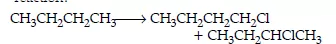27. In which of the following molecules carbon atom marked with an asterisk (*) is asymmetric?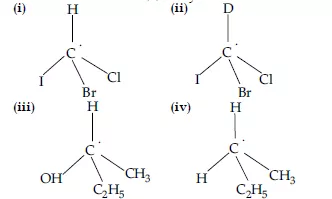(a) (i), (ii), (iii), (iv)
(b) (i), (ii), (iii)
(c) (ii), (iii), (iv)
(d) (i), (iii), (iv)

28. Which of the following structures is enantiomeric with the molecule (a) given below?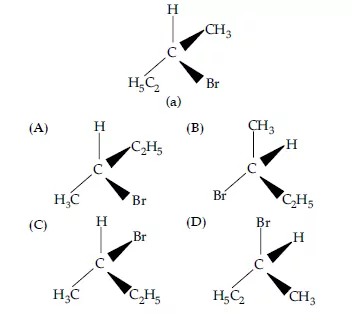29. Identify the compound Y in the following reaction.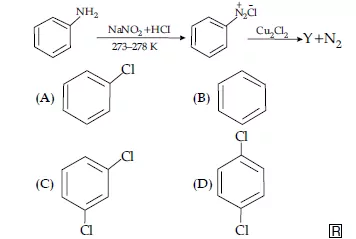30. What is ‘A’ in the following reaction?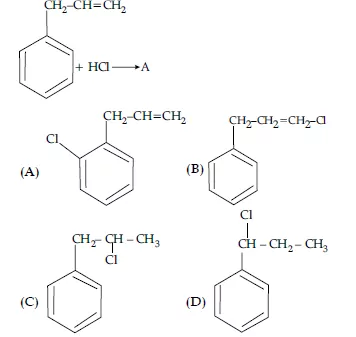The substitution reaction of alkyl halide mainly occurs by SN1 or SN2 mechanism. Whatever mechanism alkyl halides follow for the substitution reaction to occur, the polarity of the carbon halogen bond is responsible for these substitution reactions. The rate of SN1 reactions is governed by the stability of carbocation whereas, for SN2reactions, the steric factor is the deciding factor. If the starting material is a chiral compound, we may end up with an inverted product or racemic mixture depending upon the type of mechanism followed by alkyl halide. Cleavage of ethers with HI is also governed by steric factor and stability of carbocation, which indicates that in organic chemistry these two major factors help us in deciding the kind of product formed.
The following questions are multiple-choice questions. Choose the most appropriate answer
(i) Which of the following alkyl halides will undergo SN1 reaction most readily
(a) (CH3)3C-F
(b) (CH3)3C-Cl
(c) (CH3)3C-Br
(d) (CH3)3C-I

(ii) Alkyl halides undergoing nucleophilic bimolecular substitution involve
(a) Retention of configuration
(b) Racemic mixture
(c) Inversion of configuration
(d) Formation of carbocation

(iii) Which of the following has a chiral carbon
(a) 1-Bromo-2,2-dimethylpropane
(b) 2-Bromo-2-methylpropane
(c) 2-Bromo-2-methyl butane
(d) 1-Bromo-2-methyl butane

(iv) Reaction of C6H5CHBr with aqueous sodium hydroxide follows
(a) SN1 mechanism
(b) SN2 mechanism
(c) Any of the above two depending upon the temperature of the reaction
(d) Saytzeff rule

32. On distilling phenol with Zn dust, one gets
(a) Toluene
(b) Benzaldehyde + ZnO
(c) ZnO + benzene
(d) Benzoic acid

33. To distinguish between salicylic acid and phenol, one can use
(a) NaHCO3 solution
(b) 5% NaOH solution
(c) Neutral FeCl3
(d) bromine water

Chemistry Practice MCQs for Class 12

To get answers click the post

CHEMISTRY PRACTICE MCQS (ANSWERS) FOR CLASS 12 – CHAPTER 2, 10